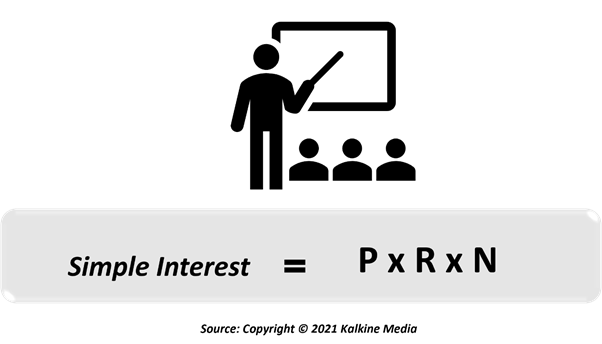# Simple Interest

• Updated on

What is Simple Interest?

Simple Interest is used to calculate interest for a principal/loan amount and does not take into consideration the compounding effect.

A borrower pays a fee to the lender for taking out a loan for a specified period of time. This borrowing cost that the borrower pays over and above the principal/loan amount for a fixed period is called simple interest.

Simple interest majorly applies to short-term loans or mortgages for biweekly proposals.

Summary
• Simple Interest is the amount charged for using borrowed money for a specified period of time.
• Simple interest is calculated on the principal amount of a deposit/loan, whereas compound interest is based on the principal amount and interest that accrues in every period of time.
• Bonds, mortgages and car loans are some examples of simple interest.
• Simple interest is more useful if an individual wants a loan for a shorter time period.

How is Simple Interest Computed?

Simple Interest is calculated by multiplying principal amount (P),  interest rate (R), and time period (t).

Principal (P): Amount borrowed initially from a bank or invested.

Interest Rate (R): Rate at which the loan or principal is given to someone for some time.

Time (T): Time is the duration for which principal amount is given to someone.

Amount (A): Amount that has to be returned, which principal (borrowed amount) plus interest.Simple Interest is paid or obtained over a certain time period. It is a fixed percentage of the principal amount that was either borrowed or lent.

The period must be expressed for the same time duration as the rate. If a rate of interest is given at a yearly rate like 6%, then the time period must also be expressed in years.

For example: If a person takes a loan of Rs 20,000 from a bank for a year and the rate of interest is 5% p.a. What amount and interest they will have to pay at year end?

Here the loan sum is Rs 20,000 (P), ROI is 5% (R) and the time period (T) is one year. Simple Interest is (20,000 x 5 x 1) /100 equals to 1000. The total amount to be paid by the person will be 20,000 plus 1000 as interest. He will pay 21,000 in total at the year end.

How is Simple Interest different from Compound Interest?

Simple interest is only levied on the amount borrowed/loaned. The more money that is borrowed/lent for a longer time, the more will be the simple interest.

Compound Interest is the interest computed on principal and interest amount accumulated over the previous periods. It is the money a person makes apart from the simple interest income from their invested capital.

Most banks make use of compound interest as they can receive more money in the form of interest from their clients.

What are some of the real applications of simple interest?

Simple interest keeps the total amount that a borrower needs to pay to be lesser than compound interest. Some of the real-life applications where simple interest is used are bonds, mortgages, etc.

Bonds pay an interest which is not compounding in the form of coupon payments. Loans that make use of simple interest are better for the borrower than loans that make use of compound interest. Another example is a certificate of deposit, a type of bank investment that pays an agreed money amount after a certain date.

A car loan is also one example of simple interest as it is amortised every month as the outstanding loan balance falls every month with monthly payments and the same happens with the interest.

What are the pros and cons of simple interest?

Simple interest can prove to be beneficial for people who want to take a loan if the person chooses to pay back principal amount with simple interest. Subsequently, the total amount owed by that person will be the interest computed on the initial loan amount or principal.

Simple interest is more useful if an individual needs a loan for a short time period like 1 month or 6 months. It is often computed on a daily basis and is majorly advantageous for individuals who make loan payments on time or early maybe every month.

However, if an individual wants to grow their money, a simple interest account must not be considered. A compound interest account in contrast shows better results for money growth than a simple interest account. There can also be many other costs factored into loan than just interest, which might affect the total loan amount but may not be added in interest rate.

##### Top ASX Listed Companies

Definition:
We use cookies to ensure that we give you the best experience on our website. If you continue to use this site we will assume that you are happy with it. OK